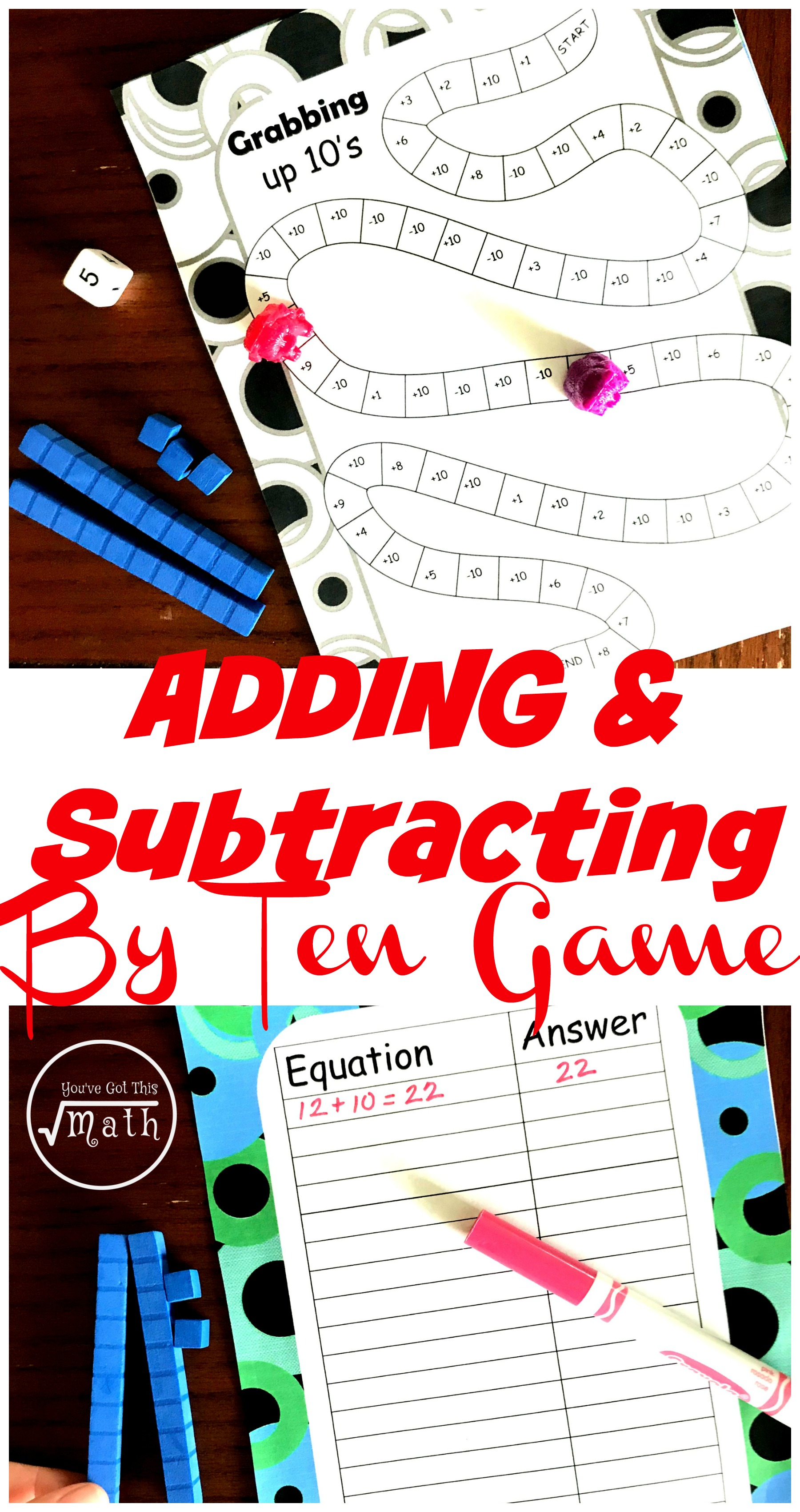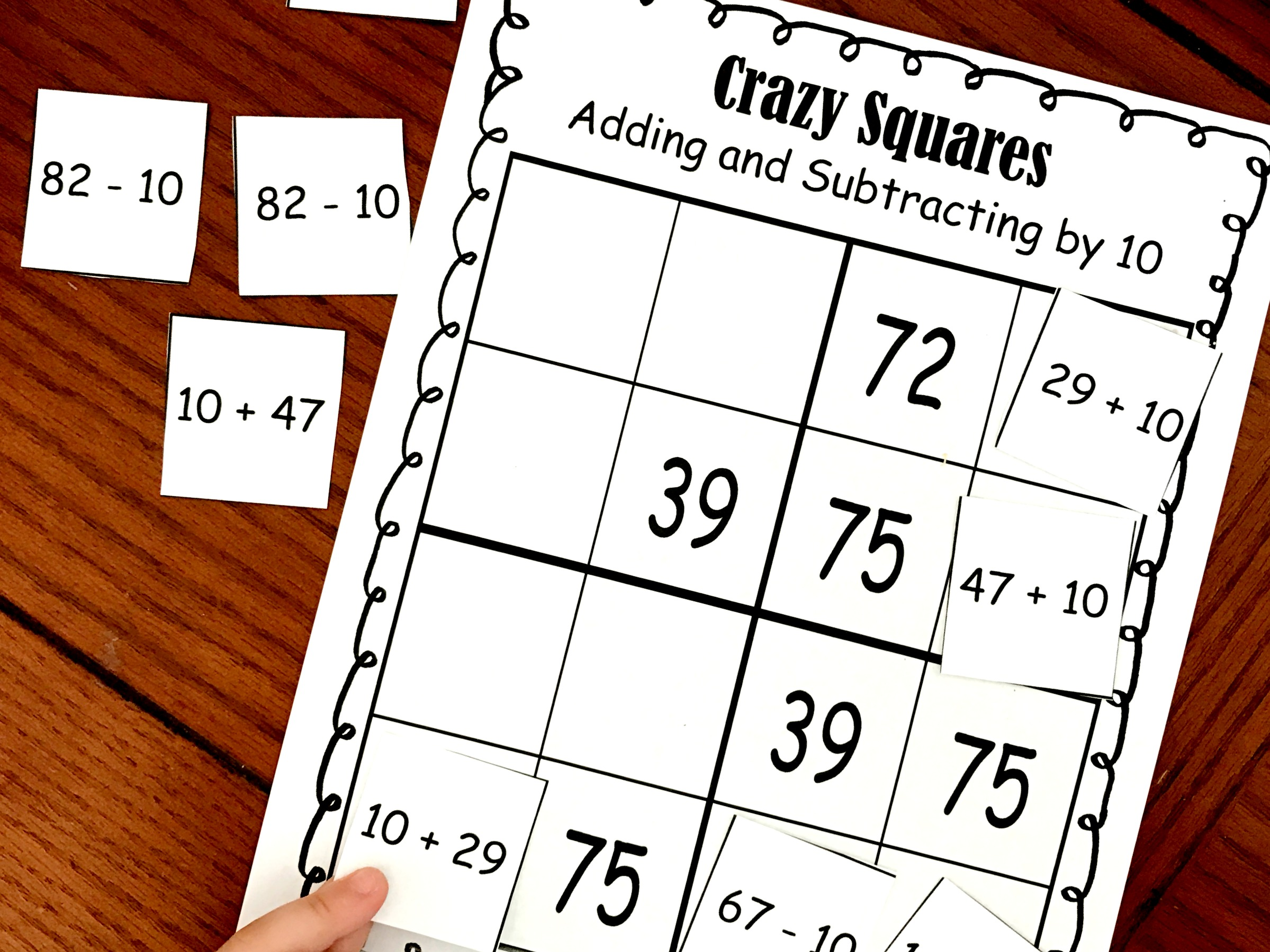Home » Math » FREE Hands-on Adding 10 Game Using Base Ten Blocks

# FREE Hands-on Adding 10 Game Using Base Ten Blocks

This free adding 10 game has children adding and subtracting by 10 and adding and subtracting by one using base ten blocks.Adding and subtracting by 10’s is important work. It builds place value sense and paves the way for metal math even when adding two two-digit numbers.

I have spent a lot of time working on this with my two oldest. My favorite way to teach this is by using the hundreds chart. Whether they are solving number puzzles or solving addition and subtraction problems, the hundreds chart is an easy way to see what happens when we add or subtract 10.

(We simply move up or down, and the number in the tens place does not change)

Today though, we are moving beyond the hundreds chart to using base ten blocks. And we are going to explore this new method of adding and subtracting by 10 through an adding 10 game.

## Prep-Work

• First, print off game board, record sheet, and base ten blocks if you need them.
• Next, laminate the recording sheet or place in a sheet protector.
• Finally, gather up game markers, dry erase markers, a die, and base ten blocks.

## Adding 10 Game Rules:

The game begins with the children gathering up 30 tens or 3 longs.

Once this is done, player one rolls and moves up that many spaces. The player then adds or subtracts base ten blocks based on what the square says. They then write an equation on their record sheet to show what they did.

### Example:For example, the player had 12 and then rolled an add 10. First, they would add 1 long. Now they can see that they have 22.

Next they write down what they did. 12 + 10 = 22

## Finishing up the Game:

Players take turns rolling, adding and subtracting, and writing equations until both players reach the end.

The player with the largest number wins.

This adding 10 game is a simple way for kiddos to practice this important skill! I hope you enjoy it as much as we did!

You’ve Got This,

Rachel

You May Also Like:

Adding and Subtracting by 10 Logic ProblemsAdding by Multiples of 10 using the Hundreds Chart and Manipulatives